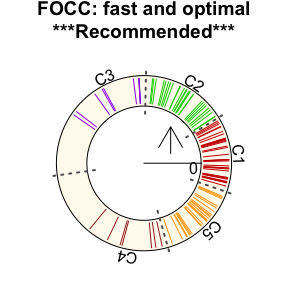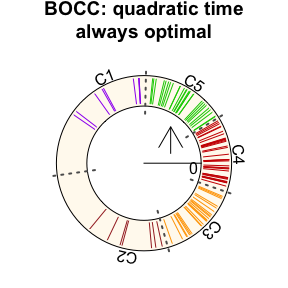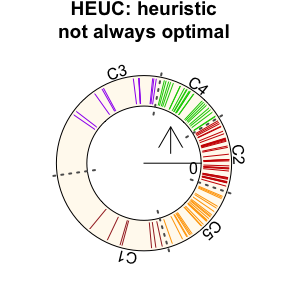# Tutorial on optimal circular clustering

#### Updated: 2021-07-27; 2020-12-12; 2020-11-29; 2020-09-05. Created: 2020-08-07

The fast optimal circular clustering (FOCC) (Debnath and Song 2021) algorithm can efficiently and optimally cluster circular data. Here circular data broadly refer to data points on any non-self-intersecting loop. We demonstrate the main functions offered by the OptCirClust package.

## Data preparation

Circular datasets can be obtained from different sources, including simulated data and real data like circular genomes. Here we generate simulated circular data. The input circular $$O$$ contains some points belonging to a circle with a Circumference=6. We will find $$K=5$$ clusters in this data.

library(OptCirClust)

O = rnorm(100)
K = 5
Circumference = 6

## Clustering the circular data

Now we demonstrate how to cluster the data using three different algorithms: the recommended fast optimal circular clustering (FOCC), the slow brute force optimal circular clustering (BOCC), and the slow and heuristic circular clustering (HEUC). The BOCC is implemented by extending the linear clustering algorithm Ckmeans.1d.dp in the identically named package. The HEUC is implemented by extending the heuristic $$K$$-means algorithm offered by the kmeans function in R. We set the random seed to be one so that the same result will be generated by the HEUC method.

# Our recommended method is the fast and optimal FOCC:
result_FOCC <- CirClust(O, K, Circumference, method = "FOCC")

# The slow and optimal BOCC:
result_BOCC <- CirClust(O, K, Circumference, method = "BOCC")

# The slow and heuristic HEUC:
result_HEUC <- CirClust(O, K, Circumference, method = "HEUC")

## Visualizing circular clusterings

The clustering outcomes obtained from the CirClust function can be visualized using the plot function.

opar <- par(mar=c(0,0,2,0))

plot(result_FOCC, main = "FOCC: fast and optimal\n***Recommended***")plot(result_BOCC, main = "BOCC: quadratic time\nalways optimal")plot(result_HEUC, main = "HEUC: heuristic\nnot always optimal")par(opar)

The circle represents the circular coordinate system with the origin marked by horizontal line and the $$0$$. The lines on the circle are the data points and the dashed line represents the cluster borders. The radial line from the center to the inner circular border along with $$0$$ shows the origin of the circular coordinate system. The arrow shows the coordinate direction. Points belonging to a cluster are marked with identical color. Clusters are numbered according to the cluster number produced by the corresponding algorithm.

The clustering outcomes can be used for further analysis of the circular data.